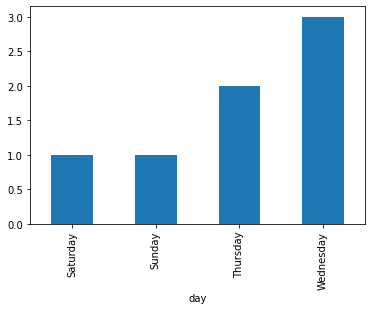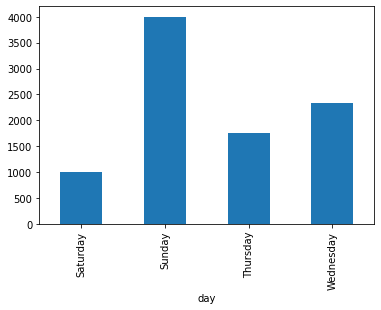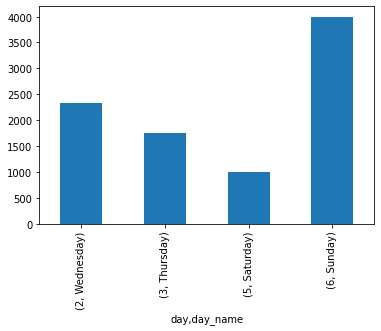Looking to create a Bar Plot per day of week based on dates in Pandas?

If so, I'll show you the steps to create a simple DataFrame with dates and plot bar chart per day of week.

## Step 1: Create example Pandas DataFrame

To start, let's create simple DataFrame which has dates like:

``````data = {'productivity': [80, 20, 60, 30, 50, 55, 95],
'salary': [3500, 1500, 2000, 1000, 2000, 1500, 4000],
'age': [25, 30, 40, 35, 20, 40, 22],
'duedate': ["2020-10-14", "2020-10-15","2020-10-15", "2020-10-17","2020-10-14","2020-10-14","2020-10-18"],
}
data_ix = ['Tim', 'Jim', 'Kim', 'Bim', 'Dim', 'Sim', 'Lim']
df = pd.DataFrame(data, index=data_ix)
``````

data:

productivity salary person duedate
0 80 3500 Tim 2020-10-14
1 20 1500 Jim 2020-10-15
2 60 2000 Kim 2020-10-15
3 30 1000 Bim 2020-10-17
4 50 2000 Dim 2020-10-14
5 55 1500 Sim 2020-10-14
6 95 4000 Lim 2020-10-18

Note: In this case, `duedate` is created as string - which restrict usage of date methods like:

`.dt.date`

If you try to use them like:

`df['duedate'].dt.date`

You will get error like:

`AttributeError: Can only use .dt accessor with datetimelike values`

## Step 2: Convert Column to DateTime in Pandas

Next steps is to convert `duedate` into DateTime column by:

``````df['duedate'] =  pd.to_datetime(df['duedate'])
``````

or giving the format:

``````df['duedate'] =  pd.to_datetime(df['duedate'], format='%d%b%Y:%H:%M:%S.%f')
``````

## Step 3: Create new column Day of Week

Once you have DataFrame with correct data and types you can create new column which have date of week based on a date in the same row:

``````df['day'] = df['duedate'].dt.day_name()
``````

Now DateFrame should look like:

productivity salary person duedate day
0 80 3500 Tim 2020-10-14 00:00:00 Wednesday
1 20 1500 Jim 2020-10-15 00:00:00 Thursday
2 60 2000 Kim 2020-10-15 00:00:00 Thursday
3 30 1000 Bim 2020-10-17 00:00:00 Saturday
4 50 2000 Dim 2020-10-14 00:00:00 Wednesday
5 55 1500 Sim 2020-10-14 00:00:00 Wednesday
6 95 4000 Lim 2020-10-18 00:00:00 Sunday

Note: If you like to use the number instead of the name of the day you can use:

`df['day'] = df['duedate'].dt.weekday`

## Step 4: Plotting Dates and Bar Plots - day of week

The final step is to plot Bar chart based on day of week by which can be done in Python and Pandas by:

``````df[['day', 'person']].groupby('day').count().plot(kind='bar', legend=None)
``````

Which looks like to:If you like to plot numeric data and use `mean` or `sum` instead of `count`:

``````df[['day', 'salary']].groupby('day').mean().plot(kind='bar', legend=None)
``````

output:## Step 5: Sort Bar Plot Week Days

If you need to sort the week days in bar plot chart in Python like:

• Monday
• Tuesday
• Wednesday

You can do a simple trick:

• convert days to names and numbers
• use both as a index
• sort the index

Below you can find the solution:

``````df['day'] = df['duedate'].dt.dayofweek
df['day_name'] = df['duedate'].dt.day_name()

df[['day', 'salary', 'day_name']].groupby(['day', 'day_name']).mean().sort_index().plot(kind='bar', legend=None)
``````

The result is bar plot with day of week sorted in natural order: